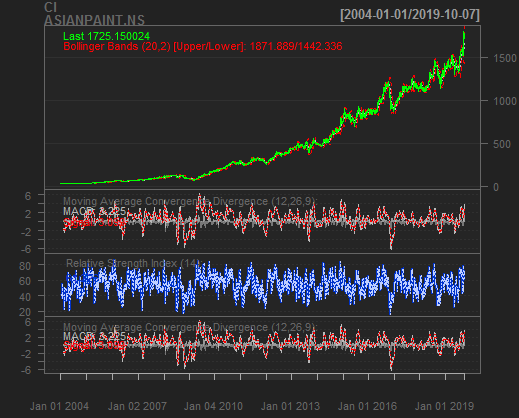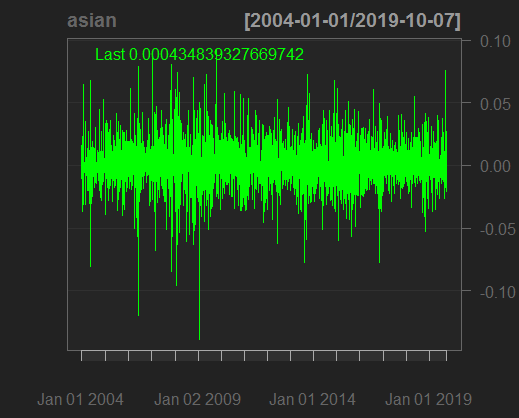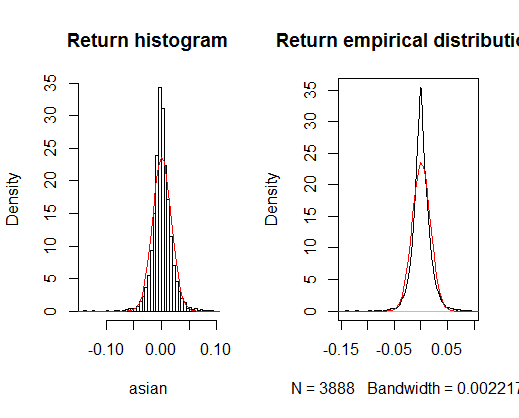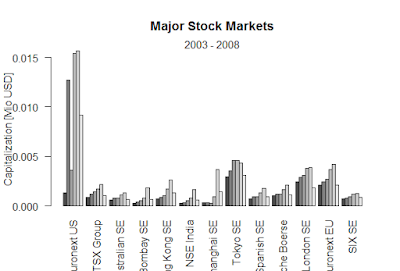Showing posts with label Stock-price-asianpaints. Show all posts
Showing posts with label Stock-price-asianpaints. Show all posts

## Stock price Asian paints-R

The stock price of asian paint data has been taken from yahoo finance.com ,we have find ,how to add macd,rsi,bbands.
> getSymbols("ASIANPAINT.NS", from="2004-01-01", to=Sys.Date())
‘getSymbols’ currently uses auto.assign=TRUE by default, but will
 "ASIANPAINT.NS"
Warning message:
ASIANPAINT.NS contains missing values. Some functions will not work if objects contain missing values in the middle of the series. Consider using na.omit(), na.approx(), na.fill(), etc to remove or replace them.
> chartSeries(Cl(ASIANPAINT.NS))ASIANPAINT.NS

> asian <- dailyReturn(Cl(ASIANPAINT.NS), type='log')
Warning message:
In to_period(xx, period = on.opts[[period]], ...) :
missing values removed from data
> chartSeries(asian)ASIANPAINT.NS

> par(mfrow=c(2,2))
> acf(asian, main="Return ACF");
> pacf(asian, main="Return PACF");
> acf(asian^2, main="Squared return ACF");
> pacf(asian^2, main="Squared return PACF")ASIANPAINT.NS

> par(mfrow=c(1,1))
> m=mean(ret);s=sd(asia);
> m=mean(asian);s=sd(asian);
> par(mfrow=c(1,2))
> hist(asian, nclass=40, freq=FALSE, main='Return histogram');curve(dnorm(x,
+ mean=m,sd=s), from = -0.3, to = 0.2, add=TRUE, col="red")
> plot(density(asian), main='Return empirical distribution');curve(dnorm(x,
+ mean=m,sd=s), from = -0.3, to = 0.2, add=TRUE, col="red")ASIANPAINT.NS

> par(mfrow=c(1,1))
> kurtosis(asian)
daily.returns
7.620324
> plot(density(asian), main='Return EDF - upper tail', xlim = c(0.1, 0.2),
+      ylim=c(0,2))
> curve(dnorm(x, mean=m,sd=s), from = -0.3, to = 0.2, add=TRUE, col="red")
> plot(density(asian), xlim=c(-5*s,5*s),log='y', main='Density on log-scale')
> curve(dnorm(x, mean=m,sd=s), from=-5*s, to=5*s, log="y", add=TRUE,

+       col="red")ASIANPAINT.NS

Conclusion
> qqnorm(asian);qqline(asian);
> garch11.spec = ugarchspec(variance.model = list(model="sGARCH",
+ garchOrder=c(1,1)), mean.model = list(armaOrder=c(0,0)))
> asian.garch11.fit = ugarchfit(spec=garch11.spec, data=asian)
> coef(asian.garch11.fit)
mu        omega       alpha1        beta1
1.179234e-03 4.167129e-05 1.501916e-01 7.081935e-01
> vcov(asian.garch11.fit)
[,1]          [,2]          [,3]
[1,]  5.894881e-08  3.591573e-11  2.688488e-07
[2,]  3.591573e-11  7.195746e-11  1.279425e-07
[3,]  2.688488e-07  1.279425e-07  4.897634e-04
[4,] -3.569481e-07 -3.714889e-07 -8.477845e-04
[,4]
[1,] -3.569481e-07
[2,] -3.714889e-07
[3,] -8.477845e-04

[4,]  2.089896e-03
> infocriteria(asian.garch11.fit)

Akaike       -5.415942
Bayes        -5.409496
Shibata      -5.415944

Hannan-Quinn -5.413654
> uncmean(asian.garch11.fit)
 0.001179234
> uncvariance(asian.garch11.fit)

 0.0002942577

### Black-Scholes formula-R

Black-Scholes formula-R > BlackScholes <- function(TypeFlag = c("c", "p"), S, X, Time, r, b, sigma) { TypeFla...